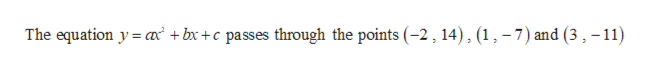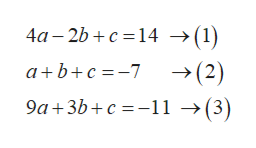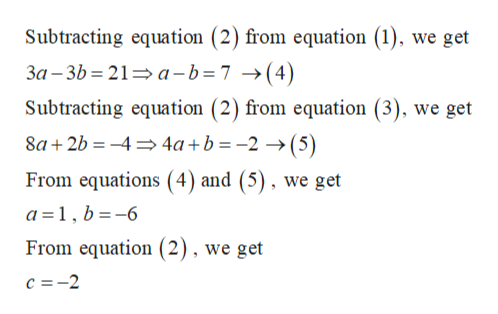Find the quadratic function y ax +bx+ c whose graph passes through the given points.(-2,14), (1,-7), (3,-11)-0y =Enter your answer in the answer box.O IOneDriveTake a Test ...HP Nurse2DesktopThis PCacerFBFTF6F5F4F3F2F1Esc432UYTREWTabLOLL

Questionhelp_outlineImage TranscriptioncloseFind the quadratic function y ax +bx+ c whose graph passes through the given points. (-2,14), (1,-7), (3,-11) -0 y = Enter your answer in the answer box. O I OneDrive Take a Test ... HP Nurse2 Desktop This PC acer FB FT F6 F5 F4 F3 F2 F1 Esc 4 3 2 U Y T R E W Tab LO LL fullscreen
Step 1

Given that,help_outlineImage TranscriptioncloseThe equation y a bxc passes through the points (-2 ,14), (1, -7) and (3, -11) fullscreen
Step 2

Substituting the points in the equation, we gethelp_outlineImage Transcriptionclose4a 2bc 14 ->(1) a b+c-7-»(2) 9a+3b+c -11 -»(3) fullscreen
Step 3

Solving these equations , ...help_outlineImage TranscriptioncloseSubtracting equation (2) from equation (1), we get 3a -3b 21 a-b=7 ->(4) Subtracting equation (2) from equation (3), we get 8a2b4 4a+b =-2 >(5) From equations (4) and (5), we get a 1, b-6 From equation (2), we get c 2 fullscreen

Want to see the full answer?

See Solution

Want to see this answer and more?

Our solutions are written by experts, many with advanced degrees, and available 24/7

See Solution
Tagged in

Equations and In-equations